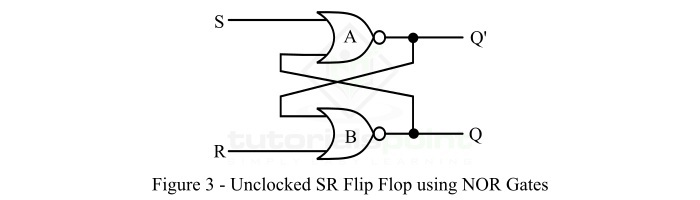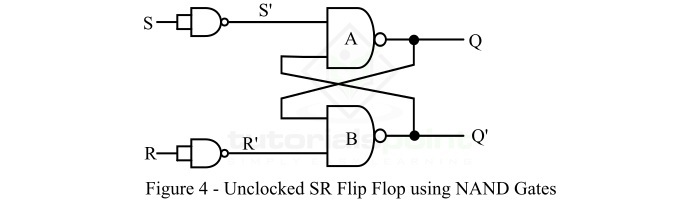# Asynchronous or Unclocked S-R flip-flop

## What is S-R Flip-Flop?

In digital electronics, the S-R flip-flop is a sequential logic circuit that have two inputs, i.e. S and R, and two outputs, i.e. Q and Q'.

The input S represent "Set" and the input R represents "Reset". The output Q is the normal output of the flip-flop, and Q' is the inverted output of the flip-flop, it is the complement of the normal output Q of the flip-flop. The S-R flip flop is a one-bit storage device used in several digital electronics systems.

The basic block diagram of an S-R flip-flop is shown in Figure-1.Based on the presence or absence of clock signal, S-R flip-flop can be classified into two types namely, synchronous S-R flip-flop and asynchronous S-R flip-flop. The synchronous S-R flip-flop is one which generates output only when clock signal is activated. Hence, it is also known as clocked S-R flip-flop.

On the other hand, an asynchronous S-R flip-flop is one whose output is available at any time the inputs are applied to the circuit. Asynchronous S-R flip flop is also known as unclocked S-R flip-flop because its output is not controlled by the clock signal, but only controlled by inputs applied.

As the title of this article implies, here we shall only talk about asynchronous or unclocked S-R flip flop.

## What is Asynchronous S-R Flip Flop?

An asynchronous S-R flip flop, also called unclocked S-R flip-flop or simply S-R latch, is a sequential logic circuit which is used to store one bit binary information. It has two inputs and two outputs. The inputs are S (represents "Set" input) and R (represents "Reset" input) of the flip-flop. The first output is Q which is called normal output of the flip-flop, and another output is Q', which is called complemented output.

The block diagram of an asynchronous or unclocked S-R flip-flop is shown in Figure-2.The unclocked or asynchronous S-R flip flop can be constructed by using two cross coupled NOR gates or NAND gates.

Let us now discuss each construction (using NOR gates and using NAND gates) of the S-R flip-flop along with their operation and truth table.

## Asynchronous S-R Flip-Flop using NOR Gates

As mentioned earlier, the unclocked S-R flip flop can be constructed by using two cross-coupled NOR gates as shown in Figure-3.It has two inputs namely: S (Set) and R (Reset), and two outputs namely: Q (Normal output) and Q' (Inverted output).

This asynchronous S-R flip flop for different possible combinations of inputs S and R operates as described as below −

• When S = 0 and R = 0 − In this case, the inputs to both NOR gates are logic 0. Hence, there is no effect on the output of the circuit. As a result, the outputs remain in their previous states. This condition of the unclocked S-R flip flop is called "No Change" or "Hold Condition".

• When S = 1 and R = 0 − In this case, the output of the NOR gate A will become zero because the input S is logic 1. As a result, both inputs of the NOR gate B become logic 0, and thus, the output of the NOR gate B is logic 1 making Q equal to 1. This is called "set condition" of the unclocked S-R flip flop.

• When S = 0 and R = 1 − In this case, the output of the NOR gate B will become zero because the input R is logic 1, i.e. Q = 0. As a result, both inputs of the NOR gate A become logic 0, and thus, the output of the NOR gate A is logic 1 making Q' equal to 1 and Q = 0. This is called "reset condition" of the unclocked S-R flip-flop.

• When S = 1 and R = 1 − This is called "forbidden condition or invalid state" of the S-R flip-flop, because this combination of inputs forces the output of both NOR gates to become logic 0, which is not possible.

The truth table of unclocked S-R flip flop based on the above described operation is given below.

SRQDescription
00NCNo Change
010Reset
101Set
11XForbidden

## Asynchronous S-R Flip-Flop using NAND Gates

We can also implement the unclocked S-R flip-flop by using two cross coupled NAND gates. The asynchronous S-R flip flop using NAND gates is shown in Figure-4 below.It can be seen that the asynchronous S-R flip flop is an active low input S-R flip flop. Now, let us discuss the working of the asynchronous SR flip flop for different possible input combinations.

• When S = 0 and R = 0, i.e. S' = 1 and R' = 1 − In this case both inputs i.e. S' and R' are Logic 1, thus the output remains in previous state. This is called hold state of the S-R flip flop.

• When S = 0 and R = 1, i.e. S' = 1 and R' = 0 − In this case, the input S' is Logic 1 and input R' is Logic 0. Thus, the output of the NAND gate B will become logic 1. Therefore, the output of the NAND gate A, i.e. Q becomes logic 1. This is called reset state of the flip flop.

• When S = 1 and R = 0, i.e. S' = 0 and R' = 1 − In this case, the input S' is logic 0 and input R' is logic 1. As the input S' is logic 0, thus the output of the NAND gate A, i.e. Q becomes logic 1. This is called set state of the S-R flip flop.

• When S = 1 and R = 1, i.e. S' = 0 and R' = 0 − In this case, both inputs are logic 0, i.e., outputs of both NAND gates must be logic 1 which is not allowed because the outputs must be complement of each other. This is called forbidden or invalid state of the flip flop.

Based on this discussion, we can derive the truth table of the asynchronous S-R flip flop realized using NAND gates.

SS'RR'QDescription
0101NCNo Change
01100Reset
10011Set
1010XForbidden

This is all about Asynchronous or Unclocked S-R Flip Flop in digital electronics.

Updated on: 25-Apr-2023

553 Views